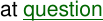Class Notes (1,100,000)
CA (630,000)
U of C (8,000)
ACCT (100)
All (30)
Lecture

ACCT 301 Lecture Notes - Historical Cost, Fixed Asset, Financial Statement

Department
Accounting
Course Code
ACCT 301
Professor
All

Page:
of 2Chapter 9
I have found this note difficult to understand and follow, perhaps due to the difficulty
of the terms used to describe this note. But who needs this kind of formality?
Simply this note says, okay you’ve revalued your assets in the past, you’ve made
some profits, some losses, you may have disposed some of them too, if that is so,
suppose that the revaluations did not take place, what would the profit or loss be if
they were based on the historical cost figure?
We may answer that question by taking the following steps:
Step 1
Find out the profit on ordinary activities before taxation.
Step 2
a) Calculate the depreciation charges of all assets based on their revaluation
figures.
b) Then calculate the depreciation charges but based on their original historical
cost figure.
c) Find the difference between a) and b), and note it down.
Step 3
Were there any disposals of F.A that have been previously revalued? (There must be
or else, this note wouldn’t be needed in the first place).
a) Well, if the answer is yes, we should calculate the profits/losses made on the
revalued figure. E.g. the revalued cost of the F.A is 200,000, and the sales
proceeds are 300,000. Then the profit would be 100,000. Simple Right?!
b) Now we should calculate the profits/losses that would have made if we used
the asset’s historical cost figure rather than the revaluation cost. E.g. if the
Historical cost of the fixed asset was 100,000 rather than 200,000 (revalued
amount), then the profit would be 200,000. Get it?!
c) We will now simply calculate their difference; in this case the difference
between the two profits is 200 less 100, which are 100,000.
Step 4
we now simply add up the figures obtained in step 2c) and 3c) and the profit before
tax to obtain the historical cost profit on ordinary activities before taxation.
FRS 3, requires that we note down the steps taken from 1 to 4 in a way of note to be
disclosed in the financial statements, and this should be done as per the following
format:
Reconciliation of Profit to Historical Cost Profit
For the year ending 31 December 20x3
\$’000
Reported profit on ordinary activities before taxation Step 1
Realization of property revaluation gains Step 3
Differences between historical cost depreciation charge
and the actual depreciation charge of the period calculated
on revalued amounts. Step 2
Historical cost profit on ordinary activities before taxation xxx
now, we know why we do it, how to do it, but the next question is why did we do it
this way?
Step 1
Profits before tax were used because taxation levels may vary from year to year.
Step 2
it is known that the larger the depreciation charges, the lower the profit before tax
would be, since depreciation is an ‘operating expense that is deducted before arriving
at the profit before tax.
As such, revaluing an asset means that, the depreciation charge based on the
revalued amount would change, and thus, affecting the value of the net profit before
tax.
Therefore, to arrive at the Historical cost profit, one must reconcile the current profit
before tax, with any changes of depreciation charged due to revaluations.
This is done, by finding the differences between the historical based depreciation
charges and the revaluation based depreciation charges. Their difference is added
back to the net profit before tax, (if the revaluation depreciation charges are larger
than the historical ones), or subtracted from the net profit, (if the revaluation
depreciation charges are lower than the historical ones.)
Step 3
once an asset is revalued, the difference between the historical cost and the revalued
figure is transferred to the Revaluation Reserve. If at a later period, the asset is
disposed of, any profits or losses would be calculating the difference between the
book value of the asset (Revaluation figure less accumulated depreciation), and the
Sales proceeds.
Thus, the amounts transferred to revaluation reserve remain untouched.
If however, the asset remained at its historical cost figure, rather than revalued, the
profit or loss on its disposal would be the book value (historical cost less accumulated
depreciation), and the sales proceeds.
If an asset was revalued upwards, the profit or loss on the asset would be much lower,
and the opposite is correct if the asset was revalued downwards. This profit or loss, is
transferred to the P&L A/C, which in turn increases or decreases the net profit before
tax.
Therefore, to arrive at the historical cost profit, one must follow the steps in Step 3.